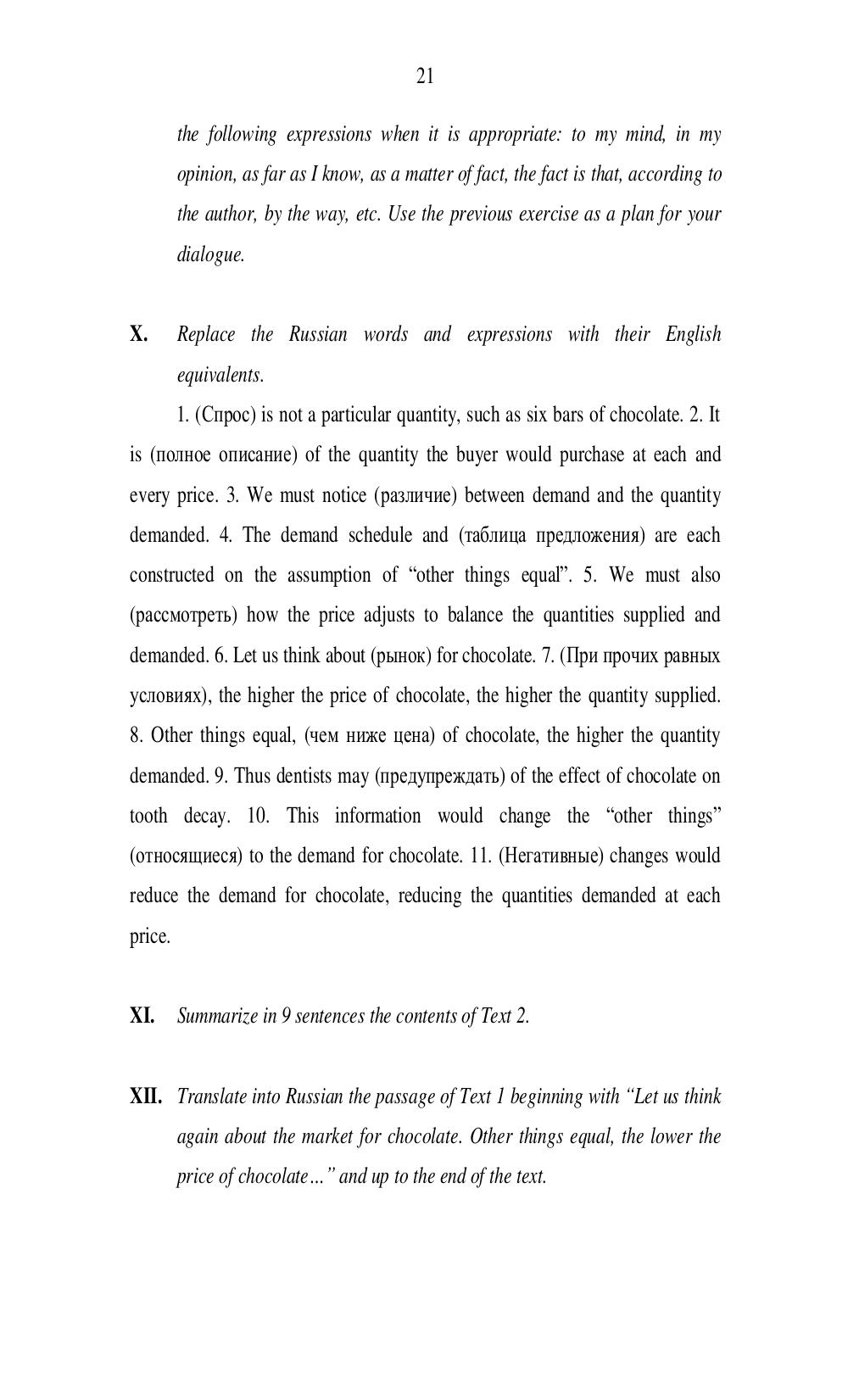# Английский язык. Агафонова И.Г - 21 стр.

Составители:

Рубрика:

• ## Иностранный язык

21
the following expressions when it is appropriate: to my mind, in my
opinion, as far as I know, as a matter of fact, the fact is that, according to
the author, by the way, etc. Use the previous exercise as a plan for your
dialogue.
X. Replace the Russian words and expressions with their English
equivalents.
1. (Спрос) is not a particular quantity, such as six bars of chocolate. 2. It
is (полное описание ) of the quantity the buyer would purchase at each and
every price. 3. We must notice (различие ) between demand and the quantity
demanded. 4. The demand schedule and (таблица предложения) are each
constructed on the assumption of other things equal. 5. We must also
(рассмотреть ) how the price adjusts to balance the quantities supplied and
demanded. 6. Let us think about (рынок) for chocolate. 7. (При прочих равных
условиях), the higher the price of chocolate, the higher the quantity supplied.
8. Other things equal, (чем ниже цена ) of chocolate, the higher the quantity
demanded. 9. Thus dentists may (предупреждать ) of the effect of chocolate on
tooth decay. 10. This information would change the other things
(относящиеся) to the demand for chocolate. 11. (Негативные ) changes would
reduce the demand for chocolate, reducing the quantities demanded at each
price.
XI. Summarize in 9 sentences the contents of Text 2.
XII. Translate into Russian the passage of Text 1 beginning with Let us think
again about the market for chocolate. Other things equal, the lower the
price of chocolate…” and up to the end of the text.21

the following expressions when it is appropriate: to my mind, in my
opinion, as far as I know, as a matter of fact, the fact is that, according to
the author, by the way, etc. Use the previous exercise as a plan for your
dialogue.

X.       Replace the Russian words and expressions with their English
equivalents.
1. (Спрос) is not a particular quantity, such as six bars of chocolate. 2. It
is (полное описание) of the quantity the buyer would purchase at each and
every price. 3. We must notice (различие) between demand and the quantity
demanded. 4. The demand schedule and (таблица предложения) are each
constructed on the assumption of “other things equal”. 5. We must also
(рассмотреть) how the price adjusts to balance the quantities supplied and
demanded. 6. Let us think about (рынок) for chocolate. 7. (При прочих равных
условиях), the higher the price of chocolate, the higher the quantity supplied.
8. Other things equal, (чем ниже цена) of chocolate, the higher the quantity
demanded. 9. Thus dentists may (предупреждать) of the effect of chocolate on
tooth decay. 10. This information would change the “other things”
(относящиеся) to the demand for chocolate. 11. (Негативные) changes would
reduce the demand for chocolate, reducing the quantities demanded at each
price.

XI.      Summarize in 9 sentences the contents of Text 2.

XII. Translate into Russian the passage of Text 1 beginning with “Let us think
again about the market for chocolate. Other things equal, the lower the
price of chocolate…” and up to the end of the text.